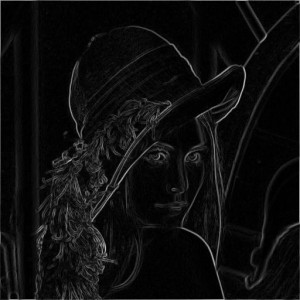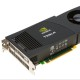# Image editing using ArrayFire: Part 2

A couple of weeks back, we did a post on a few image editing functions using ArrayFire library. Today, we shall be doing the second post in the series `Image Editing using ArrayFire`. We will be looking at the following operations today.

• Image distortion
• Noise reduction
• Edge filters
• Boundary extraction
• Difference of gaussians

Code and sample input/outputs corresponding to each operation are described below.

#### Image distortion

We will be looking at spread and pick filters in this section. Both of these filters are fundamentally the same, they replace each pixel in the original image with one of it’s neighboring pixels. How the neighbor is chosen is essentially the difference between spread and pick. Both of these functions use a common function `getRandomNeighbor` to select neighboring pixels. The code snippet for `getRandomNeighbor` is given below.

```array getRandomNeighbor(const array &in, int windW, int windH)
{
array rnd = 2.0f*randu(in.dims(0), in.dims(1))-1.0f;
array sx = seq(in.dims(0));
array sy = seq(in.dims(1));
array vx = tile(sx, 1, in.dims(1)) + floor(rnd*windW);
array vy = tile(sy.T(), in.dims(0), 1) + floor(rnd*windH);
array vxx = clamp(vx,0,in.dims(0));
array vyy = clamp(vy,0,in.dims(1));
array in2 = moddims(in, vx.elements(), 3);
return moddims(in2(vyy*in.dims(0)+vxx, span),in.dims());
}
```
```/**
* randomly pick neighbor from given window size and replace the
* current pixel with the randomly chosen color.
* No new colors are introduced, unlike hurl.
*/
array spread(const array &in, int window_width, int window_height)
{
return getRandomNeighbor(in,window_width,window_height);
}
```
```/**
* randomization - controls % of total number of pixels in the image
* that will be effected by random noise
* repeat - # of times the process is carried out on the previous steps output
*/
array pick(const array &in, int randomization, int repeat)
{
int w = in.dims(0);
int h = in.dims(1);
float f = randomization/100.0f;
int dim = (int)(f*w*h);
array ret_val = in.copy();
for(int i=0;iInput imageAfter Pick filter

We will at look hurl noise now. Despite the fancy name, what the filter essentially does is replace some of the pixels of the image with random color. New colors are introduced into the resulting image. Therefore, giving a high `repeat` input value might result in an image that is completely different from the original.
/**
* randomization - controls % of total number of pixels in the image
* that will be effected by random noise
* repeat - # of times the process is carried out on the previous steps output
*/
array hurl(const array &in, int randomization, int repeat)
{
int w = in.dims(0);
int h = in.dims(1);
float f = randomization/100.0f;
int dim = (int)(f*w*h);
array ret_val = in.copy();
array temp = moddims(ret_val,w*h,3);
for(int i=0;iInput ImageAfter adding hurl noise

Noise reduction
Noise reduction is typically achieved using smoothing filters. We will look at bilateral, median and Gaussian blur filters today. Our library has a built-in function(af::bilateral) that readily does bilateral filtering on an image.Input imageBilateral smoothingBilateral with more spatial and chromatic variance

As you can see in the above images, bilateral is not quite effective with hurl noise. Increasing spatial radius might help a little but might result in loosing features as shown in the third picture. Next, we will look at Gaussian blur.
array gaussianblur(const array &in, int window_width, int window_height, int sigma)
{
array g = gaussiankernel(window_width,window_height,sigma,sigma);
return convolve(in,g);
}Input ImageAfter applying Gaussian smoothing

Gaussian blur was able to remove noise to certain extent, but it is blurring out all important features equally and the noisy pixels participate in the convolution operation. Hence, Gaussian blur might not be a right candidate always.
array medianfilter(const array &in, int window_width, int window_height)
{
array ret_val(in.dims());
ret_val(span,span,0) = medfilt(in(span,span,0),window_width,window_height);
ret_val(span,span,1) = medfilt(in(span,span,1),window_width,window_height);
ret_val(span,span,2) = medfilt(in(span,span,2),window_width,window_height);
return ret_val;
}Input ImageAfter Median filter is applied

Median filter seems to do a good job for this type of noise.
Edge filters
We will be looking at Sobel and Prewitt filters.
void prewitt(array &mag, array &dir, const array &in)
{
float h1[] = { 1, 1, 1};
float h2[] = {-1, 0, 1};

array Gy = convolve(3, h2, 3, h1, in)/6;
array Gx = convolve(3, h1, 3, h2, in)/6;

// Find magnitude and direction
mag = hypot(Gx, Gy);
dir = atan2(Gy, Gx);
}Input ImagePrewitt Gradient

void sobel(array &mag, array &dir, const array &in)
{
float h1[] = { 1, 2,  1};
float h2[] = { 1, 0, -1};

array Gy = convolve(3, h2, 3, h1, in)/8;
array Gx = convolve(3, h1, 3, h2, in)/8;

// Find magnitude and direction
mag = hypot(Gx, Gy);
dir = atan2(Gy, Gx);
}Input ImageSobel Gradient

Boundary extraction
The following code snippet extracts boundaries in an image using erosion morphological operator.
/**
* dimensions of the mask control the thickness of the boundary that
* will be extracted by the following function
*/
array boundary(const array &in, const array &mask)
{
array ret_val = in - erode(in,mask);
normalizeImage(ret_val);
return ret_val;
}Input ImageBoundaries

Difference of gaussians
{
array ret_val;
array g1 = gaussiankernel(w1,w1);
array g2 = gaussiankernel(w2,w2);
ret_val = (convolve(in,g1)-convolve(in,g2));
normalizeImage(ret_val);
return ret_val;
}Input ImageDifference of Gaussians

Last two sections use a utility function `normalizeImage` that basically re-scales the pixels values to [0-255] range.
void normalizeImage(array &in)
{
float min = af::min(in);
float max = af::max(in);
in = 255.0f*((in-min)/(max-min));
}

Conclusion
All the functions from this post are updated and available through github repository located here. Hope, you guys are enjoying this series.

```

1. Pingback: ArrayFire for image editing: Part 2

2.Hi,
Does the Bilateral Filter is the classic implementation or any “Approximation” for speedup (Such as prebuilt LUT)?

Moreover,
There are many people there into such things to follow you.

1.Hi Royi,

We do not use Look Up Tables for bilateral filter. As for Goolge+, we are working internally to get a verified account. This will take us a few days to set up. Please follow us on @ArrayFireBlog:disqus until that point.

1.Hi,
Could you say something about the implementation?
Which method (Article???) was implemented?
Are results the same as the classic bilateral filter?

1.2.3.4.Hi, I’m confusing with ‘ convolve(3, h2, 3, h1, in)/8; ‘
Where is the prototype of the convolve which takes 5 arguments? I can’t find at ArrayFire Github repository.

1.